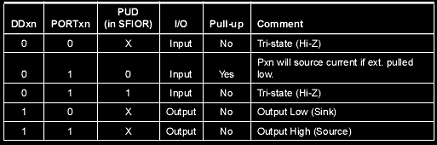# Accessing AVR microcontroller ports with AVR GCC

All AVR ports have Read-modify-write functionality when used for general I/O functionality. The direction of each separate port pin can be changed. Each pin buffer has the symmetric capability with the ability to drive and sink source. The pin driver is strong enough to drive LED directly, but it is not recommended to drive even small loads without using proper driver circuit such as transistors. All port pins have internal selectable pull-up resistors. And finally, all pins have protection diodes to both VCC and GND rails.

Each port of AVR consists of three registers DDRx, PORTx and PINx (where x means port letter). DDRx register selects the direction of port pins. If logic one is written to DDRx then the port is configured to be as output. Zero means that the port is configured as an input. If DDRx is written zero and PORTx is written logic “1” then the port is configured as input with an internal pull-up resistor. Otherwise, if PORTx is written to zero, then the port is configured as input but pins are set to tri-state and you might need to connect external pull-up resistors.

If PORTx is written to logic “1” and DDRx is set to “1”, then the port pin is driven high. And if PORTx=0, then the port is driven low.How we can control AVR port pins using C language? When using AVR GCC first we need to set up proper library where PORT register names have their addresses defined. The main library where general-purpose registers are defined is io.h located in avr directory of AVR GCC installation location.

 `#include `

Now we can use port names instead of their addresses. For instance, if we want to set all pins of PORTD as output we simply write:

 `DDRD=0xFF; ``//set port D pins as outputs`

Now we can output a number to port D:

 1 `PORTD=0x0F; ``//port pins will be set to 00001111`

if we have 8-bit variable i, we can assign this variable to port register like this:

 `uint8_t i=0x54;` `PORTD=i;`

 `DDRD=0; ``//set all port D pins as input` `i=PIND; ``//read all 8 pin bits of port Dand store to variable i`

There is the ability to access separate port pins. So all eight port pins can be used for multiple purposes.

Some of the pins may be configured as outputs and some as inputs and perform different functions.

Let’s say we need 0,2,4,6 pins to be as input and 1,3,5,7 as output. Then we do this:

 `DDRD=0; ``//reset all bits to zero` `DDRD |=(1<<1)|(1<<3)|(1<<5)|(1<<7); ``//using bit shift “<<”operation and logical OR to set bits 1,3,5,7 to “1”`

So we can output values to 1,3,5 and 7 pins

 `PORTD |=(1<<1)|(1<<3)|(1<<5)|(1<<7);`

Or clear them

 `PORTD &=~((1<<1)|(1<<3)|(1<<5)|(1<<7));`

Reading of port pins is easy. Set any pin(s) for input like this:

 `DDRD &=~((1<<1)|(1<<3)); ``//This clears bits 1 and 3 of port direction register` `i=PIND; ``//reads all 8 pins of port D`

You can read 1 and 3 bits by using masks or simply shift i value by 1 ar 3 positions to compare LSB with 1. Of course, there are some functions in sfr_defs.h library like bit_is_set() or bit_is_clear() to check particular bits and make these tasks little easier.

Following example should clarify some issues:

 `#include "avr\io.h"` `#include "avr\iom8.h"` `int` `main(``void``) {` `  ``DDRD&=~_BV(0);``//set PORTD pin0 to zero as input` `  ``PORTD|=_BV(0);``//Enable pull up` `  ``PORTD|=_BV(1);``//led OFF` `  ``DDRD|=_BV(1);``//set PORTD pin1 to one as output` `  ``while``(1) {` `   ``if` `(bit_is_clear(PIND, 0))``//if button is pressed` `        ``{` `            ``PORTD&=~_BV(1);``//led ON` `            ``loop_until_bit_is_set(PIND, 0);``//LED ON while Button is pressd` `            ``PORTD|=_BV(1);``//led OFF` `        ``}` `    ``}` `}`